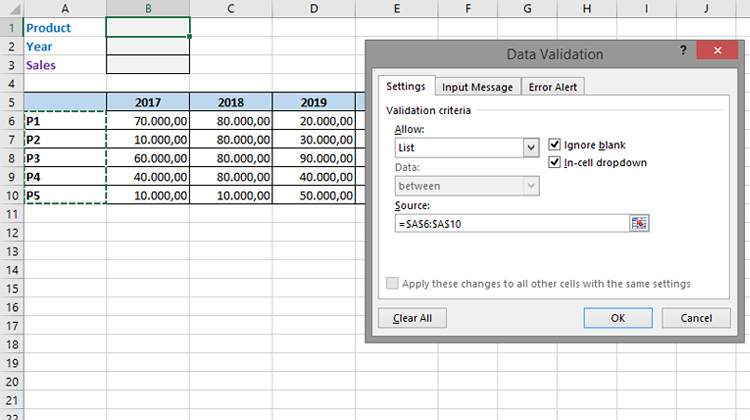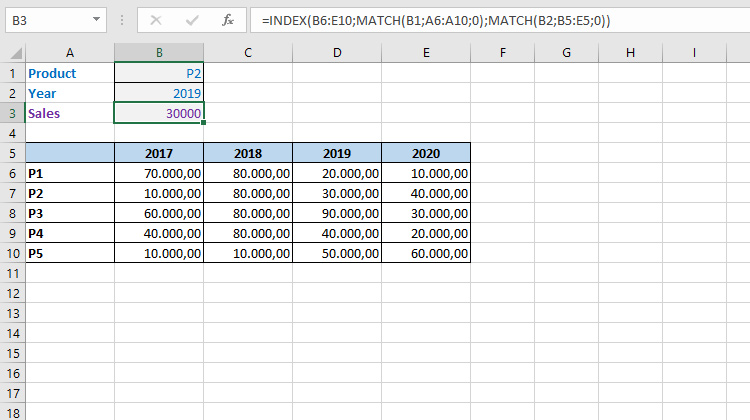# Simple sales analysis

By combining INDEX and MATCH, we can easily calculate sales results if the data we analyse is given in a table that is a matrix. By using Data Validation for certain cells you’ll get a very simple sales analysis tool that is easily scalable and can be used in more complex scenarios. Pivot tables are an irreplaceable tool but there are situations where we get similar results by a different procedure, this is one of them!

We created one simple spreadsheet with two headers. On the left are product names, and at the top of the year when the sale took place. The sections show the quantities sold. How, in an easy way, to get sales value for the selected product and the given year?

We will first set, by applying the Data Validation option, to enter the data into cells B1 and B2 by selecting from the list. The first list represents the product names (A6: A10) and the second year (B5: E5).Sales are data that are in the cross section of the product and year. Therefore, it is necessary for us to calculate the position in a row for the given data. In the case of products, this information is obtained on the basis of the formula:

=MATCH(B1;A6:A10;0)

The value of cell B1 is obtained by choosing from the product list. The selected product is searched within the name range and the MATCH function returns the position of the product. Similarly, we will write a formula for finding the selected year in a row:

=MATCH(B2;B5:E5;0)

Since the intersection of these two results contains the value of sales for its calculation we need to write the following statement:

=INDEX(B6:E10;MATCH(B1;A6:A10;0);MATCH(B2;B5:E5;0))

Each time we choose a different product or a year, a different sales value will be displayed.